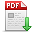# 张志刚,马新旋,王才东,等.基于三次样条插值的几何精确曲梁单元[J].郑州大学学报(工学版),2023,44(06):61-67.[doi:10. 13705/ j. issn. 1671-6833. 2023. 03. 002] 　ZHANG Zhigang,MA Xinxuan,WANG Caidong,et al.A Geometrically Exact Curved Beam Element Based on Cubic Spline Interpolation[J].Journal of Zhengzhou University (Engineering Science),2023,44(06):61-67.[doi:10. 13705/ j. issn. 1671-6833. 2023. 03. 002] 点击复制 基于三次样条插值的几何精确曲梁单元() 分享到： var jiathis_config = { data_track_clickback: true };

44卷

2023年06期

61-67

2023-09-25

## 文章信息/Info

Title:
A Geometrically Exact Curved Beam Element Based on Cubic Spline Interpolation

Author(s):
Henan Key Laboratory of Intelligent Manufacturing of Mechanical Equipment, Zhengzhou University of Light Industry, Zhengzhou 450002, China

Keywords:
DOI:
10. 13705/ j. issn. 1671-6833. 2023. 03. 002

A

Abstract:
To accurately model and simulate the multibody system including large deformation beams, a 2D large deformation curved beam element, which had few element parameters and can be integrated with the CAD geometric model, was proposed based on cubic spline interpolation. Firstly,by taking the position vector at each node and the axial position gradient vector at the two-end nodes as the global parameters, the motion of the beam centroid line was approximated using the cubic spline interpolation. Secondly, on the basis of fully considering the deformation of the slender beam, the rotation of the beam cross-section was determined by the centroid tangent according to the deformation assumption of Euler-Bernoulli beam. Finally, the axial strain and curvature of the beam were derived based on the geometrically exact beam theory, the mass matrix, nodal force and generalized external force of the planar curved beam element were derived based on the virtual power principle, and the tangent stiffness matrix was obtained. Compared with the existing large deformation planar beam element, the number of the global parameters of the proposed beam element was greatly reduced. In addition, because the vertical relationship between the beam cross-section and the tangent direction of the centroid line was guaranteed in the proposed coupling deformation field, the shear locking phenomenon in the application could be avoided. In the numerical example, through the calculation and comparison of typical examples including the static geometric nonlinear problems and dynamic rigid flexible coupling problems, it was shown that the calculation efficiency of the proposed geometrically exact curved beam element could be greatly improved while not losing the calculation accuracy.